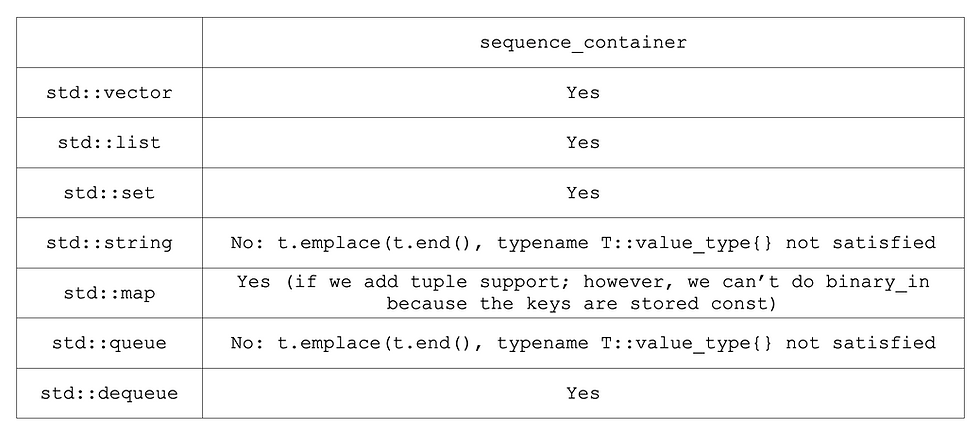top of page
• Hunter Allen

# Simplifying partial template specialization with C++20 concepts

A highly anticipated change to the C++ standard, concepts, was finally added with the C++20 standard after initially being proposed for the C++14 standard.

Concepts aim to simplify many of the painful issues that arise from the use of templates in many common scenarios, such as the inability to list requirements for template arguments, severe readability issues, and even a way to conditionally omit member functions such as constructors based on attributes that may be found in a given template argument.

One such scenario that frequently comes into play is partial template specialization. In this blog post, we will look at how partial template specialization is performed in C++17 and earlier, then we will look at the new possibilities afforded to us with the introduction of C++20’s concepts.

## SFINAE: Partial specialization without concepts

A very common situation that frequently arises when writing containers is partial specialization: the developer wishes to define a function that applies only to a certain class of template arguments. Our goal is then to give the compiler instructions to enable certain code conditionally, based on the template argument.

Suppose, for example, we’re writing functions that will serialize/deserialize data in a particular way.

template<class T> size_t binary_out(std::ostream & stream, const T & t); template<class T> size_t binary_in(std::istream & stream, T & t);

In order to do so, we could specialize T for each type that we are serializing, but doing so can be extremely repetitive for many common types. So, it might be convenient to write a case for all situations where T is Plain Old Data (POD).

In C++14, that looks like this:

template<class T, typename _> std::size_t binary_out(std::ostream & stream, const T & t); template<class T, typename _> std::size_t binary_in(std::istream & stream, T & t); template< class T, typename std::enable_if< std::is_pod< typename std::remove_cv<T>::type>::value>::type * = nullptr> inline std::size_t binary_out(std::ostream & stream, const T & t) { stream.write(reinterpret_cast<const char *>(&t), sizeof(t)); return sizeof(t); } template< class T, typename std::enable_if< std::is_pod< typename std::remove_cv<T>::type>::value>::type * = nullptr> std::size_t binary_in(std::istream & stream, T & t) { stream.read(reinterpret_cast<char *>(&t), sizeof(t)); return sizeof(t); }

This is where a partial specialization is really nice because we can specialize for an entire class of common types. The syntax we used here, however, is pretty clunky. Especially when we extend this for sets of containers, such as std::vector and std::list.

We need a few definitions to allow us to determine if the template is a sequence container.

template<class T> struct is_sequence_container : public std::false_type {}; template<class U> struct is_sequence_container<std::vector<U>> : public std::true_type {}; template<class U> struct is_sequence_container<std::list<U>> : public std::true_type {};

Then we use the same SFINAE pattern as before.

template< class T, typename std::enable_if< is_sequence_container<T>::value>::type * = nullptr> inline std::size_t binary_out(std::ostream & stream, const T & t) { size_t bytes_written = binary_out(stream, t.size()); for (const auto & element : t) { bytes_written += binary_out(stream, element); } return bytes_written; } template< class T, typename std::enable_if< is_sequence_container<T>::value>::type * = nullptr> inline std::size_t binary_in(std::istream & stream, T & t) { typename T::size_type elements; std::size_t bytes_read = binary_in(stream, elements); for (typename T::size_type to_read = elements; to_read-- > 0; ) { typename T::value_type element; bytes_read += binary_in(stream, element); t.emplace_back(std::move(element)); } return bytes_read; }

While we have been able to achieve our goal, there are several issues with this solution. The syntax is extremely clunky and hard to read, it is extremely difficult (if not impossible) to explicitly list all requirements to make a piece of code function, compiler errors are long and nearly unreadable, and, for cases like the is_sequence_containercondition we wrote, this isn’t great for writing a library, as the user must explicitly label something a container (and may not do so correctly!).

## Concepts

Due to those pain points and more, C++20 adds concepts. The basic idea behind a concept is to provide a list of traits and requirements that a template parameter must satisfy to be applied.

To declare a concept, you can use any constexpr bool evaluated expression. For example, we can write a concept for trivial_type as follows.

template<class T> concept trivial_type = std::is_trivial<T>::value;

While this seems like we’re not conserving any effort, we get a much cleaner way to declare the binary_out for trivial types.

template<trivial_type T> inline std::size_t binary_out(std::ostream & stream, const T & t) { stream.write(reinterpret_cast<const char *>(&t), sizeof(t)); return sizeof(t); }

Now, for sequence_container, we will use the required function to declare our concept.

template<class T> concept sequence_container = requires(T t) { t.size(); typename T::iterator; typename T::value_type; t.emplace(t.end(), typename T::value_type {}); } && std::forward_iterator<typename T::iterator>;

In our requires list, we are just placing expressions that we need to be valid. In the code, we used t.size(), typename T::value_type, so we need those to exist. Additionally, we use emplace, so we need to make sure that we can call emplace with an iterator to the end, as well as an element.

Lastly, we compose that list of requirements with the extra condition that typename T::iterator satisfies the requirements for the STL-defined concept std::forward_iterator.

Here’s a list of some data structures and whether or not they are considered sequence_containers by the definition above.With that definition in place, we can write the streamer functions for sequence_containers like this.

template<sequence_container T> inline std::size_t binary_out(std::ostream & stream, const T & t) { size_t bytes_written = binary_out(stream, t.size()); for (const auto & element : t) { bytes_written += binary_out(stream, element); } return bytes_written; } template<sequence_container T> inline std::size_t binary_in(std::istream & stream, T & t) { typename T::size_type elements; std::size_t bytes_read = binary_in(stream, elements); for (typename T::size_type to_read = elements; to_read-- > 0; ) { typename T::value_type element; bytes_read += binary_in(stream, element); t.insert(t.end(), element); } return bytes_read; }

For the full implementations of the above, see this folder from the Apex.AI code snippets repository.

To summarize, using C++20’s concepts can help make code more readable and make partial template specializations more flexible than with SFINAE patterns used in C++17 and earlier.